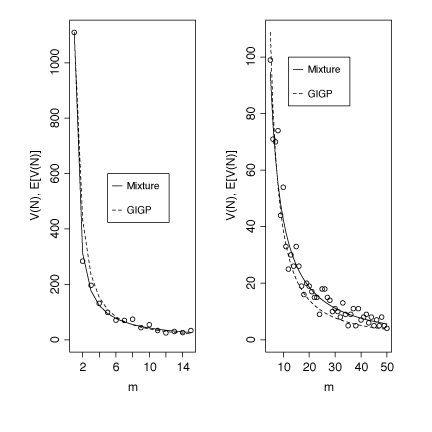## When two distributions are better than one: Mixture models and word frequency distributions.

Fiona J. Tweedie
Department of Statistics
Mathematics Building
University Gardens
GLASGOW, Scotland G12 8QW
fiona@stats.gla.ac.uk

Harald Baayen
University of Nijmegan
Max Planck Institute for Psycholinguistics
Wundtlaan 1
PB 310, 6500 AH NIJMEGEN
The Netherlands
baayen@mpi.nl

### Summary

Models for word frequency distributions are relevant for a wide range of domains of inquiry, including authorship studies, statistical language engineering, theoretical linguistics, and linguistic synergetics. For inferences based on such models to be useful, they should provide accurate descriptions of the data to which they are fitted. This paper shows that improved fits may sometimes be obtained by analysing word frequency distributions as mixtures of two or more distinct component distributions, with the gain in accuracy outweighing the increased number of model parameters.

### Introduction

Currently, there are three models for word frequency distributions available that take the dynamics of the development of spectral characteristics as a function of sample size into account: the lognormal model, the extended generalized Zipf's model, and the generalized inverse Gauss-Poisson model (GIGP), see Chitashvili and Baayen (1993), for a review of these LNRE models. Although many empirical word frequency distributions are well-described by one or more of these models, there are also word frequency distributions for which no adequate fit is available. Baayen and Tweedie (1998) discuss informally a data set concerning the frequencies of use of Dutch words with the suffix -heid (cf. -ness in English) which illustrates this point.

The word frequency distribution of -heid is problematic because the medium frequency ranges of the spectrum are more densely populated than expected by the LNRE models. This suggests that we might be dealing with a mixture of two, or more, distributions, rather than with a single homogeneous distribution. The question we have set ourselves is: Is it possible to find two component LNRE models that jointly provide an improved fit to the observed frequency spectrum of -HEID?

### Mixture Models

Mixture models describe distributions where the data can be drawn from one or more sources. Our starting point is a word frequency distribution spectrum without any indication of how it is to be decomposed into its two components. In general, when we model a word frequency spectrum we are interested in finding expected values of the elements V(m,N), the number of words occurring m times in a text of length N. The parameters of LNRE models are then chosen to make the expected value of the spectrum elements, E[V(m,N)] as close to the observed V(m,N) as possible. When a single distribution is not enough to deal with the observed data, we can consider the use of a mixture distribution, where the expected values are made up as follows:
E[V(m,N)] = E_1[V(m,pN)] + E_2[V(m,(1-p)N)],
where p is the proportion of the data coming from the first distribution, usually called the mixing parameter, and (1-p) the proportion which comes from a second distribution. E_1 and E_2 indicate the expected values under the different distributions.

It can be shown for each of the LNRE models that

E[V(m,pN)|Z,...] = p E[V(m,N)|Z/p,...]
with Z the LNRE parameter of the distribution. This general relation, which expresses a form of self-similarity in word frequency distributions, allows us to show that limiting properties of the mixture, such as its estimated population number of types, is the sum of its mixture components. Similarly, expressions of variances and covariances of the spectrum elements can be derived, so that the mixture model itself is again a complete LNRE model.

### -HEID as a Mixture Distribution

Figure 1 plots the number of types V(m,N) with frequency m in a sample of size N as a function of m, for m = 2, ..., 15 in the left panel, and for m=15, ..., 100 in the right panel, using dots (N=167353). The dashed line represents the GIGP fit to the data (\hat{Z} = 41.5554, \hat{b} = 0.00765648, \hat{\gamma} = -0.446889), which overestimates for low m and underestimates for larger m. Other LNRE models provide even worse fits to the data. The solid line represents the mixture model for a Lognormal component (\hat{Z} = 200, \hat{\sigma} = 2.05) and a GIGP component (\hat{b} = 0.000000002093, \hat{Z} = 82.9848, \hat{\gamma} = -0.565). The mixing parameter p equals 0.96. The MSE (mean squared error) for the GIGP fit is 3390.6, and X^{2}(13) = 1734.7, p < .1*10^-18. For the mixture model, the MSE is reduced to 97.1, and with X^{2}(10) = 19.58, p=0.0334 we have no reason to reject the model. We have obtained similar improvements in goodness-of-fit for other word frequency distributions that thusfar resisted adequate modeling. At the conference, we will present further examples of the advantages of using mixture models where `pure' models fail, and we will demonstrate the software that we have been developing to fit mixture LNRE models to empirical data.### References

Baayen, R. H. and Tweedie, F.J.: 1998, "Mixture models and word frequency distributions." Abstracts of the ALLC/ACH Conference, Debrecen, July 1998.

Chitashvili, R. J. and Baayen, R. H.: 1993, "Word Frequency Distributions," In: G. Altmann and L. Hrebicek, L. (Eds.), Quantitative Text Analysis, Wissenschaftlicher Verlag Trier, Trier, pp. 54-135.